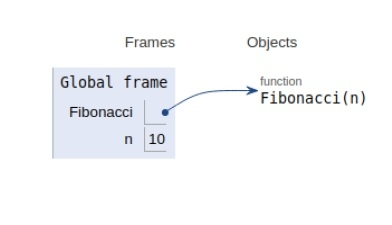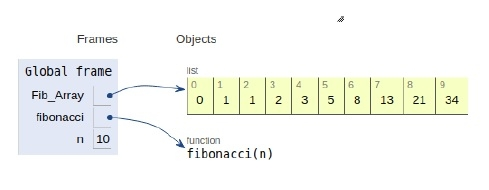# Python Program for n-th Fibonacci number

A Fibonacci number is defined by the recurrence relation given below −

Fn = Fn-1 + Fn-2

With F0= 0 and F1 = 1.

First, few Fibonacci numbers are

0,1,1,2,3,5,8,13,..................

We can compute the Fibonacci numbers using the method of recursion and dynamic programming.

Now let’s see the implementation in the form of Python script

## Approach 1: Recursion Method

#### Example

Live Demo

#recursive approach
def Fibonacci(n):
if n<0:
print("Fibbonacci can't be computed")
# First Fibonacci number
elif n==1:
return 0
# Second Fibonacci number
elif n==2:
return 1
else:
return Fibonacci(n-1)+Fibonacci(n-2)
# main
n=10
print(Fibonacci(n))

#### Output

34

The scope of all the variables declared is shown below.## Approach 2: Dynamic Programming Method

#### Example

Live Demo

#dynamic approach
Fib_Array = [0,1]
def fibonacci(n):
if n<0:
print("Fibbonacci can't be computed")
elif n<=len(Fib_Array):
return Fib_Array[n-1]
else:
temp = fibonacci(n-1)+fibonacci(n-2)
Fib_Array.append(temp)
return temp
# Driver Program
n=10
print(fibonacci(n))

#### Output

34

The scope of all the variables declared is shown below## Conclusion

In this article, we learned about the computation of nth Fibonacci number using recursion and dynamic programming approach.